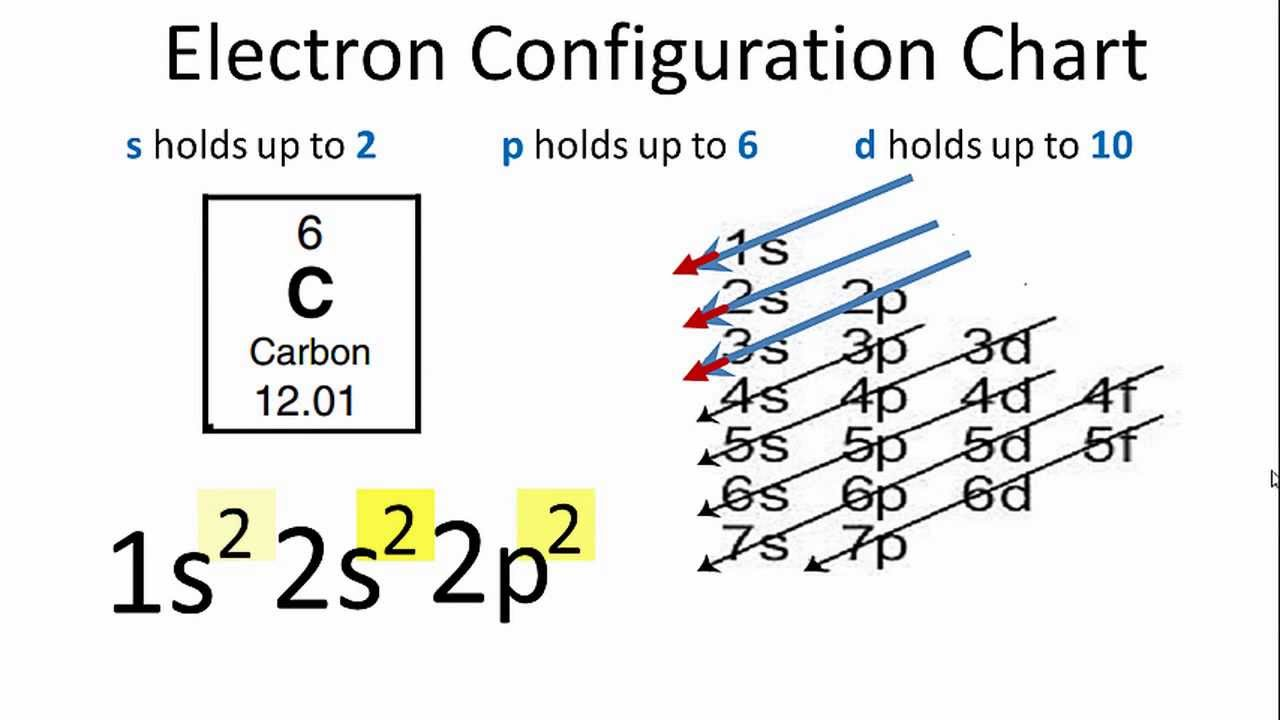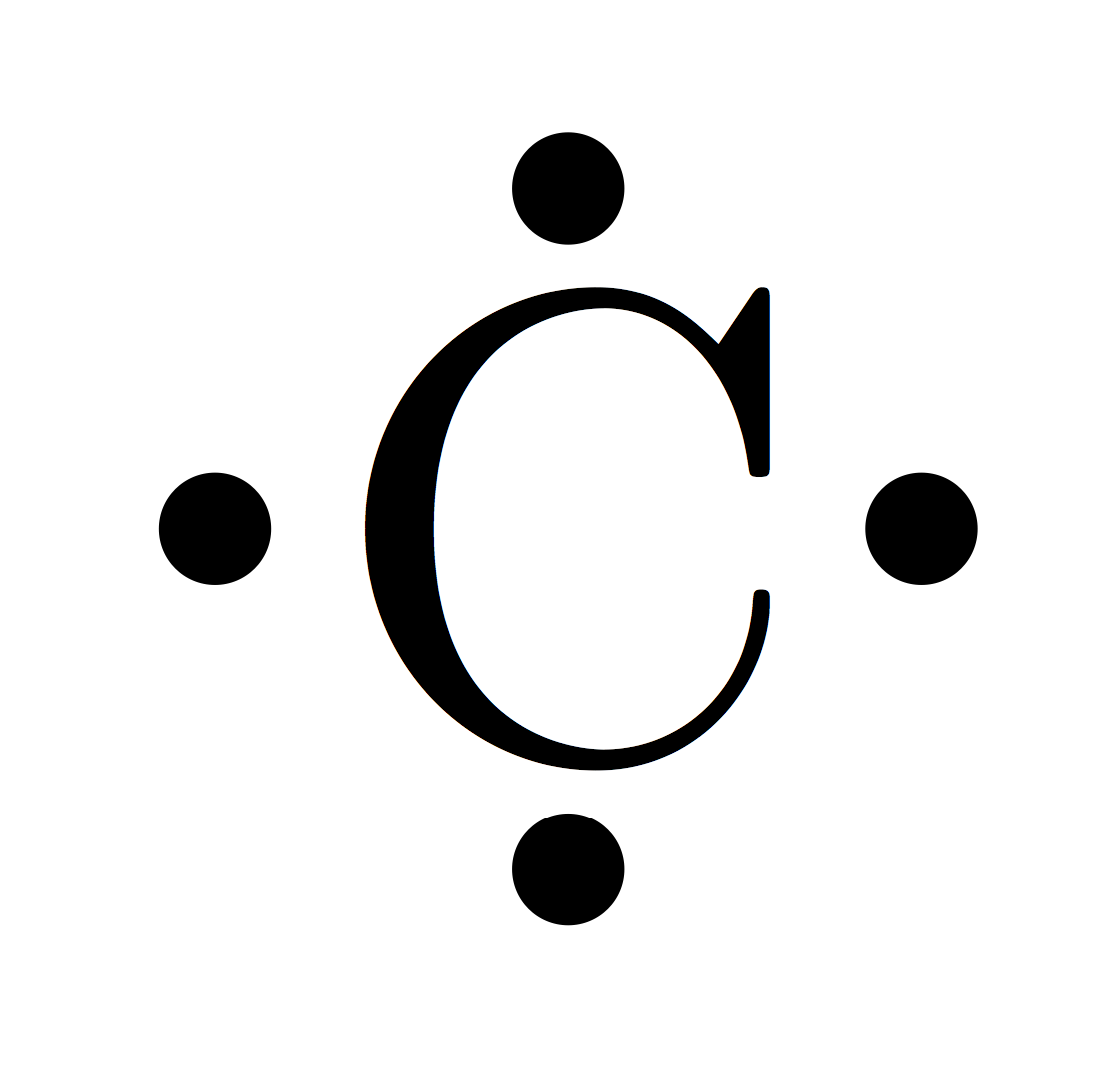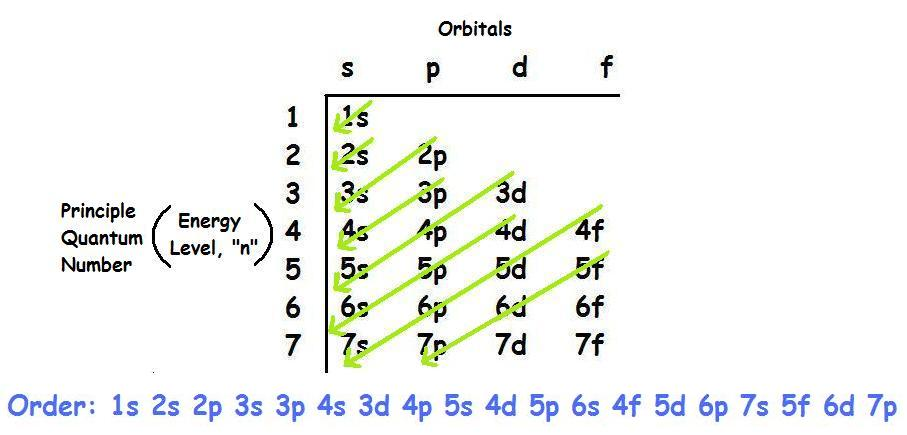# How to Resolve The Valency of Electron Configuration For Carbon

Carbon is considered to be the sixth element which has sixth electrons in the totality. In the electronic configuration of the carbon, the first two will be held by the 1s orbital, the next two in the 2s orbital, and the remaining ones will be held by the 2p orbital.### Carbon Electron Dot Diagram

Electron diagram is also known as Lewis dot diagram since it is first used by the Gilbert N. Lewis.This diagram is used as a notation to depict the numbers of electron valence in an atom. If you are seeking for the carbon electron dot diagram then here you can find the one.

#### How Do You Figure Out The Electron Configuration

As we have elaborated above that there are sixth electrons in the carbon, and in the electronic configuration of the carbon, all these electrons are taken by the orbital. This configuration can be shown as 1s22s22p2.